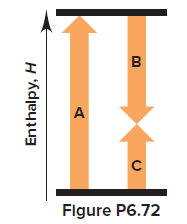# Problem: Write the balanced overall equation (equation 3) for the following process, calculate ΔHoverall, and match the number of each equation with the letter of the appropriate arrow in Figure P6.72:(1) N2 (g) + O2 (g) ⟶ 2NO (g)                Δ  H = 180.6 kJ(2) 2NO (g) + O2 (g) ⟶ 2NO2 (g)           Δ H = −114.2 kJ--------------------------------------------------------------------------------------------(3)                                                 ΔH     overall = ?

###### Problem Details

Write the balanced overall equation (equation 3) for the following process, calculate ΔHoverall, and match the number of each equation with the letter of the appropriate arrow in Figure P6.72:

(1) N2 (g) + O2 (g) ⟶ 2NO (g)                Δ  H = 180.6 kJ
(2) 2NO (g) + O2 (g) ⟶ 2NO2 (g)           Δ H = −114.2 kJ

--------------------------------------------------------------------------------------------
(3)                                                 ΔH     overall = ?